## Package overview

Overview of ggdist.

ggdist-package ggdist

Visualizations of Distributions and Uncertainty

## Point summaries and intervals

Calculate point summaries and intervals

point_interval() qi() ll() ul() hdi() Mode() hdci() mean_qi() median_qi() mode_qi() mean_ll() median_ll() mode_ll() mean_ul() median_ul() mode_ul() mean_hdi() median_hdi() mode_hdi() mean_hdci() median_hdci() mode_hdci()

Point and interval summaries for tidy data frames of draws from distributions

curve_interval()

Curvewise point and interval summaries for tidy data frames of draws from distributions

cut_cdf_qi()

Categorize values from a CDF into quantile intervals

## Visualizing sample data and distribution vectors

Stats for visualizing tidy data frames of draws (e.g. sample data, draws from a bootstrap distributation, draws from a Bayesian posterior) and distribution vectors (such as distributional objects and posterior::rvar()s).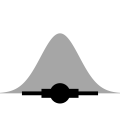stat_slabinterval()

Slab + interval plots for sample data and analytical distributions (ggplot stat)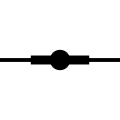stat_pointinterval()

Point + multiple-interval plot (shortcut stat)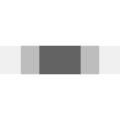stat_interval()

Multiple-interval plot (shortcut stat)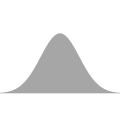stat_slab()

Slab (ridge) plot (shortcut stat)stat_halfeye()

Half-eye (density + interval) plot (shortcut stat)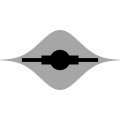stat_eye()

Eye (violin + interval) plot (shortcut stat)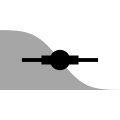stat_ccdfinterval()

CCDF bar plot (shortcut stat)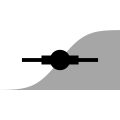stat_cdfinterval()

CDF bar plot (shortcut stat)stat_gradientinterval()

Gradient + interval plot (shortcut stat)stat_histinterval()

Histogram + interval plot (shortcut stat)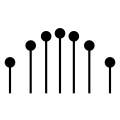stat_spike()

Spike plot (ggplot2 stat)stat_dotsinterval()

Dots + point + interval plot (shortcut stat)stat_dots()

Dot plot (shortcut stat)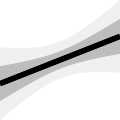stat_lineribbon()

Line + multiple-ribbon plot (shortcut stat)stat_ribbon()

Multiple-ribbon plot (shortcut stat)

Geoms for visualizing data that has already been summarized (e.g. into density function evaluations, points, intervals, etc).geom_slabinterval()

Slab + point + interval meta-geomgeom_pointinterval()

Point + multiple-interval plot (shortcut geom)geom_interval()

Multiple-interval plot (shortcut geom)geom_slab()

Slab (ridge) plot (shortcut geom)geom_spike()

Spike plot (ggplot2 geom)geom_dotsinterval()

Automatic dotplot + point + interval meta-geomgeom_dots()

Dot plot (shortcut geom)geom_weave()

Dot-weave plot (shortcut geom)geom_swarm()

Beeswarm plot (shortcut geom)geom_lineribbon()

Line + multiple-ribbon plots (ggplot geom)

## Distributions

Helper functions for manipulating and visualizing analytical distributions.

parse_dist() r_dist_name()

Parse distribution specifications into columns of a data frame

dstudent_t() pstudent_t() qstudent_t() rstudent_t()

Scaled and shifted Student's t distribution

dlkjcorr_marginal() plkjcorr_marginal() qlkjcorr_marginal() rlkjcorr_marginal()

Marginal distribution of a single correlation from an LKJ distribution

marginalize_lkjcorr()

Turn spec for LKJ distribution into spec for marginal LKJ distribution

## GGplot scales

Scales for custom aesthetics used by ggdist geoms and stats

scale_point_colour_discrete() scale_point_color_discrete() scale_point_colour_continuous() scale_point_color_continuous() scale_point_fill_discrete() scale_point_fill_continuous() scale_point_alpha_continuous() scale_point_alpha_discrete() scale_point_size_continuous() scale_point_size_discrete() scale_interval_colour_discrete() scale_interval_color_discrete() scale_interval_colour_continuous() scale_interval_color_continuous() scale_interval_alpha_continuous() scale_interval_alpha_discrete() scale_interval_size_continuous() scale_interval_size_discrete() scale_interval_linetype_discrete() scale_interval_linetype_continuous() scale_slab_colour_discrete() scale_slab_color_discrete() scale_slab_colour_continuous() scale_slab_color_continuous() scale_slab_fill_discrete() scale_slab_fill_continuous() scale_slab_alpha_continuous() scale_slab_alpha_discrete() scale_slab_size_continuous() scale_slab_size_discrete() scale_slab_linewidth_continuous() scale_slab_linewidth_discrete() scale_slab_linetype_discrete() scale_slab_linetype_continuous() scale_slab_shape_discrete() scale_slab_shape_continuous() guide_colourbar2() guide_colorbar2()

Custom ggplot scales for geom_slabinterval (and derivatives)

scale_colour_ramp_continuous() scale_color_ramp_continuous() scale_colour_ramp_discrete() scale_color_ramp_discrete() scale_fill_ramp_continuous() scale_fill_ramp_discrete()

Secondary ggplot color scale that ramps from another color

guide_rampbar()

Continuous colour ramp guide

scale_thickness_shared() scale_thickness_identity() thickness()

Scale for slab thickness

scale_side_mirrored()

Side scale for mirrored slabs

## GGplot positions

Positions for dodging geoms and stats

position_dodgejust()

Dodge overlapping objects side-to-side, preserving justification

## GGplot theme

ggdist theme for ggplot2

theme_ggdist() theme_tidybayes() facet_title_horizontal() axis_titles_bottom_left() facet_title_left_horizontal() facet_title_right_horizontal()

Simple, light ggplot2 theme for ggdist and tidybayes

## Dotplot layout algorithms

Algorithms used by geom_dotsinterval() for automatically determining nice-looking dotplot binwidths and laying out dots.

find_dotplot_binwidth()

Dynamically select a good bin width for a dotplot

bin_dots()

Bin data values using a dotplot algorithm

smooth_bounded() smooth_unbounded()

Smooth dot positions in a dotplot using a kernel density estimator ("density dotplots")

smooth_discrete() smooth_bar()

Smooth dot positions in a dotplot of discrete values ("bar dotplots")

smooth_none()

Apply no smooth to a dotplot

## Density functions

Density functions used by stat_slabinterval() and by the density smoothers for dotplot layout.

density_bounded()

Bounded density estimator using the reflection method

density_unbounded()

Unbounded density estimator

density_histogram()

Histogram density estimator

## Bandwidth estimators

Bandwidth estimators for use with density_unbounded() and density_bounded().

bandwidth_nrd0() bandwidth_nrd() bandwidth_ucv() bandwidth_bcv() bandwidth_SJ() bandwidth_dpi()

Bandwidth estimators

## Distribution bounds estimators

Distribution bounds estimators for use with density_bounded().

bounder_cdf()

Estimate bounds of a distribution using the CDF of its order statistics

bounder_cooke()

Estimate bounds of a distribution using Cooke's method

bounder_range()

Estimate bounds of a distribution using the range of the sample

## Histogram layout algorithms

Algorithms for bin selection and alignment for use with density_histogram().

breaks_fixed() breaks_Sturges() breaks_Scott() breaks_FD()

Break (bin) selection algorithms for histograms

align_none() align_boundary() align_center()

Break (bin) alignment methods

## Automatic partial functions

Overview of automatic partial function application (currying) used by several ggdist function families

automatic-partial-functions

Automatic partial function application in ggdist

## Probability expressions

Experimental mini-domain-specific language for probability expressions in stat_slabinterval() aesthetics.

Pr_() p_()

Probability expressions in ggdist aesthetics

## Weighted quantiles and CDFs

Weighted generalizations of quantile() and ecdf() used internally by several algorithms.

weighted_quantile() weighted_quantile_fun()

Weighted sample quantiles

weighted_ecdf()

Weighted empirical cumulative distribution function

## Compatibility with other packages

Functions to convert the ggdist naming scheme (for point_interval()) to and from other packages’ naming schemes.

to_broom_names() from_broom_names() to_ggmcmc_names() from_ggmcmc_names()

Translate between different tidy data frame formats for draws from distributions

## Datasets

Datasets used for examples and testing

RankCorr RankCorr_u_tau

Thinned subset of posterior sample from a Bayesian analysis of perception of correlation.

## Deprecated functions

Deprecated functions.

ggdist-deprecated StatSampleSlabinterval stat_sample_slabinterval StatDistSlabinterval stat_dist_slabinterval stat_dist_halfeye stat_dist_eye stat_dist_ccdfinterval stat_dist_cdfinterval stat_dist_gradientinterval stat_dist_slab stat_dist_pointinterval stat_dist_interval stat_dist_lineribbon stat_dist_dotsinterval stat_dist_dots

Deprecated functions and arguments in ggdist